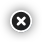# Anschutz Speedometer Chronograph

\$ 273.95
Qty:

### Anschutz Speedometer Chronograph

Features:

• Measuring range 6.1 - = 500 m / s
• Deviation <-1% @ 250 m / s
• Current consumption: 100mA (with LCD backlight)
• Power supply: 2x AA Alkaline battery or AC / DC converter ~ 230V / 7 -12V
• Dimensions 135 x 67 x 87 mm
• Weight 600 g

Function:

• Velocity v [m / s]
• The kinetic energy E [J]
• Shot meter
• Mean kinetic energy Esr [J]
• Average speed Vsr [m / s]
• Minimum speed Vmin [m / s]
• Maximum speed Vmax [m / s]
• Absolute speed DVSR = [Vmax-Vmin]
• Standard deviation SV [m / s]
• Speed feet per second V [fps]
• Rate of fire RoF (Fire speed)

Other Functions:

• Setting the weight of the ball 0.01 - 25.00 g
• Calibration (set distance between the sensors)
• Memory: 250 readings
• Transmission via RS232 or USB-COM cable to the computer